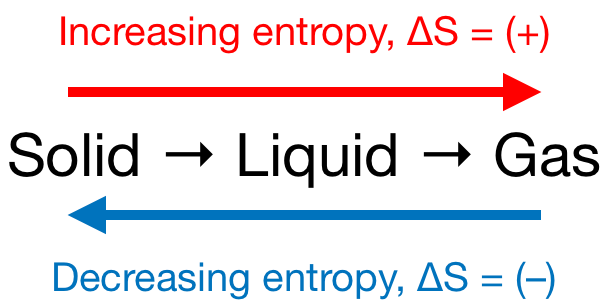Chemistry Practice Problems Entropy Practice Problems Solution: Which of the following processes have a ΔS &gt; 0?...

🤓 Based on our data, we think this question is relevant for Professor Van Der Sluys' class at PSU.

# Solution: Which of the following processes have a ΔS &gt; 0?a. 2NH3(g) + CO2(g) → NH2CONH2(aq) + H2O(l)b. lithium fluoride forms from its elementsc. 2HF(g) → H2(g) + F2(l)d. potassium iodide dissolves in pure water

###### Problem

Which of the following processes have a ΔS > 0?

a. 2NH3(g) + CO2(g) → NH2CONH2(aq) + H2O(l)

b. lithium fluoride forms from its elements

c. 2HF(g) → H2(g) + F2(l)

d. potassium iodide dissolves in pure water

###### Solution

We’re asked to determine which of the following processes have a ΔS > 0.

Also, recall that if:

• ΔS > 0 or ΔS = (+); entropy is increased

• ΔS < 0 or ΔS = (–); entropy is decreased

Remember that phase changes lead to a change in entropy:Also, recall that:

• An increase in the number of gas molecules in the reaction also leads to an increase in entropy.
• If bonds are broken then the entropy of a reaction increases,
• But, if bonds are formed then the entropy of a reaction decreases.View Complete Written Solution

Entropy

Entropy

#### Q. Given the values of ΔH∘rxn, ΔS∘rxn, and T below, determine ΔSuniv. a) ΔH∘rxn = 87 kJ , ΔS∘rxn = 146 J/K , T= 296 K, will this be spontaneous or not s...

Solved • Tue Mar 20 2018 12:24:40 GMT-0400 (EDT)

Entropy

#### Q. Arrange these gases in order of decreasing standard molar entropy: Rank from largest to smallest. Cl2 SO3 Kr

Solved • Mon Mar 19 2018 16:31:55 GMT-0400 (EDT)

Entropy

#### Q. Given the values of ΔH∘rxn, ΔS∘rxn, and T below, determine ΔSuniv. a. ΔH∘rxn = 84 kJ , ΔS∘rxn = 144 J/K , T = 303 K b. ΔH∘rxn = 84 kJ , ΔS∘rxn = 144...

Solved • Wed Mar 07 2018 10:40:01 GMT-0500 (EST)

Entropy

#### Q. Calculate ΔSfus and ΔSvap for Na.

Solved • Fri Mar 02 2018 13:21:37 GMT-0500 (EST)